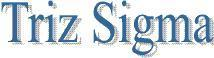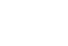Anova : Analysis of Variance online advertising online advertising Introduction ANOVA will tell me whether I have sufficient evidence to say that measurements from at least one lab differ significantly from at least one other. Here is the example problems As a manager, you want to know diference between productivity of your three subordinates. You collect data for 15 days for each subordinate. And, want to know the difference in their productivity. As a chicken nutritionist, you need reliable measures of lasalocid sodium levels in chicken feed, so you decide to test the analytical labs.You send a sample of feed containing X mg/kg lasalocid sodium to each of three independent labs, and get back the results. Anova can be used to understand the variaton in results. ANOVA measures two sources of variation in the data and compares their relative sizes. variation BETWEEN groups -for each data value look at the difference between its group mean and the overall mean variation WITHIN groups - for each data value we look at the difference between that value and the mean of its group Assumptions Samples are randomly and independently drawn Populations are normally distributed Populations have equal variances Anova Hypothesis H0: There is no significant difference among the results provided by these three laboratories/ productivity of subordinates. H1: At least one of these laboratories/subordinate provides results significantly different from at least one other. Anova Calculation One-Way ANOVA - Total VariationOne-Way ANOVA -Among groups VariationOne-Way ANOVA -Within groups VariationMSA= (SSA /(C-1)), c= Number of group MSW = (SSW /(n-c)) , n = Total number of observations in all groups Test Statistics F = MSA /MSW = Between/Within R (square) = (SSA / (SSA+SSW)) If p value is <0.05, there is significant difference between means of groups.